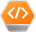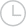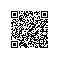# 这个字符串语法分析始终不健壮，请大家帮忙看下？400 请求出错  -问答-阿里云开发者社区-阿里云

## 这个字符串语法分析始终不健壮，请大家帮忙看下？400 请求出错kun坤2020-05-25 20:37:3169

a='[' [b=2, c=3] --> 与第一个 [ 匹配的 ] 中间的子串是： [b=2, c=3]

a='\'[ab []' [b=2, c=3] --> 与第一个 [ 匹配的 ] 中间的子串是： [b=2, c=3]

a=1 [b=2, c=3, d='\']' ] --> 与第一个 [ 匹配的 ] 中间的子串是：[b=2, c=3, d='\']' ]

``````public static String[] parseString( String str )
{
StringBuilder buf = new StringBuilder(str);
int nstr_len = buf.length();

// 标志一个<:> 是否出现在''单引号引用中，如果出现在引用中，则忽略
boolean inQuote = false;
for (int indx = 0; indx < nstr_len; indx++)
{
char c = buf.charAt(indx);
// 进入''引用中遍历
if (inQuote)
{
// 本次''引用结束
if ('\'' == c)
{
inQuote = false;
}
}
else if ('\'' == c)
{
inQuote = true;
}
// 找到合法的左括号 [
else if( '[' == c )
{
// 查找和这个合法左括号 [ 相匹配的右括号 ] 的位置
int endIndex = findMatchEndIndex(buf, indx);
// 从当前字符串中截取[]中的子串
String subStr = buf.substring(indx, endIndex+1);
// 将当前字符串中被截取的位置清空
buf.replace(indx, endIndex+1, "");

// 字符串整体长度进行缩减，保证for循环正确
nstr_len -= subStr.length();
}
}

return list.toArray(new String[list.size()]);
}

private static int findMatchEndIndex( CharSequence buf, int startIndex )
{
int buf_len = buf.length();
boolean inQuote = false;
int indx = startIndex + "[".length();
int count = 0;
for( ; indx < buf_len; indx++ )
{
char c = buf.charAt(indx);
// 进入''引用中遍历
if (inQuote) {
// 本次''引用结束
if ('\'' == c)
{
inQuote = false;
}
}
else if ('\'' == c) {
inQuote = true;
}
else if('[' == c) {
count++;
}
else if( ']' == c )
{
count--;
}
// 通过配对出现计数来获取匹配给定 [ 的右括号 ] 位置
if( count < 0 )
{
return indx;
}
}

return -1;
}
``````

上面的代码核心是处理 ' ' 单引号，采用单引号配对来标志 [ ] 是否出现在单引号内，如果在单引号内，则忽略。取消 提交回答

• kun坤2020-05-26 11:12:17

"这不典型的Stack应用吗? 楼主还在犹豫什么呢??######回复 <a href=""http://my.oschina.net/u/147822"" class=""referer"" target=""_blank"">@Grrrr : 不太明白，这种方式能拿到符合要求的 [ ] 中间的字符串信息吗？######回复 <a href=""http://my.oschina.net/jsan"" class=""referer"" target=""_blank"">@JSON : 哎```楼主可以变通下嘛~~把 ' 也看做成对出现的不就可以了,只不过不进行转义.. 也就是Stack里面只存 ' 和 [ ] 这三种符号.######我看下Stack，很多用来判断括号等是否成对出现的，这个我上面的代码也支持，但是如果判断一个 [ 是否出现在 ' ' 单引号中就比较难了。######愿闻其详，我还真没有用过Stack######啊？没有人能帮忙的吗？######不知道你这是要干嘛######我做的一个功能解析器，提取符合语法定义[ ] 中的字符串内容######正则表达式看一下######找 [ ] 配对匹配比较容易，不论是stack实现，还是计数实现都是可以的，但是关键是：要判断 [ ] 是否出现在 单引号 ' ' 中就比较难了。######

stack + deterministic state machine

一种比较简单是实现是“递归下降回溯”法。楼主参考《编译原理实践》（大概是这个名儿）中，词法解析的一个例子 —— 用“递归下降回溯法“编写算术表达式的解析和计算。

大致如下：

parse_exp()

parse_square_exp()

parse_quote_exp()

然后根据目前字符决定函数，互相调用。

######错了。是”递归下降分析“，应该没有回溯这一步。######
```public static void main(String[] args) {
String t1 = " a='\'[ab []' [b=2, c=3]";
String [] t1_arg = t1.split("");
boolean isQuat = false;
int bn = -1;
int en = 0;
int strIndex = 0;
for (String s : t1_arg)
{

if (s.equals("'") || s.equals("\'"))
{
isQuat = !isQuat;
}
if(!isQuat && s.equals("[") && bn < 0)
{
bn = strIndex;
}
if(!isQuat && s.equals("]") )
{
en = strIndex;
}
strIndex ++;
}
System.out.println(t1.substring(bn-1));
}```

我写了个 就一个循环，试了你当前给的那4个字符，你看看行不行######s.equals("\'") 这个不会成立，因为是转义字符，所以，不会有 \' 出现， \' java 认为是 '######

语法解析，首先要保证语法是没有歧义的；

这个例子就有些诡异：

[ b=2, c=3 [, d=4] ]

如果是 [b=2, c=3, [d=4]] 就比较好

首先需要找到有哪些词法单元（终结符）：

标识符 ID

整数

' 单引号

\ 反斜杠

=赋值符号

[ 左中括号

] 右中括号

再看有哪些语法单元（非终结符）：

转意字符： \+单个字符

字符串： '任意字符或者转意字符'

赋值语句： 标识符 = 表达式

表达式：  字符串， 标识符， 赋值语句， 数组

数组: [ 数组成员 ，。。。]

数组成员： 赋值语句， 表达式

而分析匹配的括号，只是上面分析的一个副产品。

######语法是没有歧义的啊： 1. [ ] 可以包含任何字符，但是出现在 ' '单引号中的 [ ] 视为普通字符； 2. [ ] 可以嵌套 3. [ ] 必须配对######

#### 引用来自“李勇2”的答案

语法解析，首先要保证语法是没有歧义的；

这个例子就有些诡异：

[ b=2, c=3 [, d=4] ]

如果是 [b=2, c=3, [d=4]] 就比较好

首先需要找到有哪些词法单元（终结符）：

标识符 ID

整数

' 单引号

\ 反斜杠

=赋值符号

[ 左中括号

] 右中括号

再看有哪些语法单元（非终结符）：

转意字符： \+单个字符

字符串： '任意字符或者转意字符'

赋值语句： 标识符 = 表达式

表达式：  字符串， 标识符， 赋值语句， 数组

数组: [ 数组成员 ，。。。]

数组成员： 赋值语句， 表达式

而分析匹配的括号，只是上面分析的一个副产品。

匹配的时候可能会存在冲突， 所以就需要给这些规则定一些优先级， 在有冲突的时候，用于选择某条规则
0 0使用钉钉扫一扫加入圈子
+ 订阅